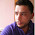Wednesday, January 2, 2013

What are the prime years in the 21st Century?

Yesterday, we checked to see if 2013 was a prime number. Because the digits sum to 2+0+1+3 = 6, we can tell immediately that it is not prime because it is divisible by 3, 2013 = 671 × 3. It turns out that 671 isn't prime, either, since it factors to 61 × 11, both of which are prime.

This means the prime factorization of 2013 = 3 × 11 × 61.  I put the primes in order from smallest to largest, but that's just me being tidy. The order of the primes doesn't matter. We say that every number has a unique prime factorization up to order.

Not everyone knows this rule for divisibility by 3, but the rule for divisibility by 5 is much simpler and better known. It shows us quickly that 2015 can't possibly be prime, since 2015 = 5 × 403. (To get the complete prime factorization, we have to check to see if 403 is prime. It turns out it isn't, 403 = 13 × 31.)

So let's ask a slightly more general problem. What numbers between 2000 and 2100 are prime? This sounds like a lot of work, which is why I decided to use Excel to answer the question.

(If you are new to Excel, you can skip down to the answer.)

Step 1: What is the square root of 2100?
In cell A1, type =sqrt(2100). The answer is 45.8257... The reason to find this is because we need to check each of our numbers for divisibility by the primes less than their square root. If a × b = n, the smaller of a and b has to be less than or equal to the square root of n and the larger must be greater than or equal to the square root of n.

Step 2: Across row 1 starting in B1, list the primes less than 45.
Starting in B1, type 2[tab] 3[tab] 5[tab] 7[tab] 11[tab] 13[tab] 17[tab] 19[tab] 23[tab] 29[tab] 31[tab] 37[tab] 41[tab] 43[tab].

Step 3: Put the numbers from 2001 to 2099 in column A.
The easiest way to do this is to type 2001 in A2, drag the value down to A101 and change the method of filling the values in to "Fill Series".

Step 4: How to tell if a number is evenly divisible by a prime.
2001/3 = 667, but 2001/5 = 400.2, which means 2001 is divisible by 3, but not by 5. The Excel formula to type will use the Excel built-in formulas IF and INT.

IF asks for a statement that is true or false, what to do if it is true and what to do if it is false.

INT takes a number x and rounds down to the largest whole number less than x. For example, =INT(2001/3) will give us 667, but =INT(2001/5) will give us 400 instead of 400.2, a difference we will be using in our logical statement to test.

Here is the formula to type into cell B2.

=IF(INT(\$A2/B\$1)=\$A2/B\$1, "yes"," ")

What this does is check if INT(2001/2) = 2001/2.  In this case it does not, so Excel will put a blank in this cell, which is what " " means.) If I click and drag this formula down the column and across the rows, The spreadsheet will now have a pattern of the word "yes" scattered about among the blank cells.

Step 5: Use an IF statement to find the numbers that weren't divisible by any of the primes we used.
We now use IF and COUNTIF in the column just beyond N to see if the word "yes" showed up in that row. If it didn't, the number in column A is prime and we will copy that number into column P.

=IF(COUNTIF(B2:N2,"yes")=0, A2, " ")

Yet again, click and drag the formula down the column. Column P now has the primes between 2000 and 2100 listed. Here they are.

2003

2011

2017

2027
2029

2039

2053

2063

2069

2081
2083

2087
2089

2099

Notice that some primes have a difference of only two, like 2081 and 2083.  These are called twin primes. Mathematicians have known since the time of Euclid that there are an infinite number of primes, which means that whatever big number n you choose, there have to be primes larger than n. It is unknown if the twin primes are infinite. It is not of vital importance, but mathematicians are fascinated by problems that are easy to state and difficult to solve.

Tomorrow: A practical application of number theory known as casting out nines.

1.2.